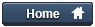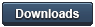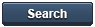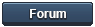### Gammon Forum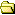Entire forum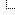Electronics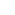Microprocessors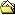Turn your Arduino into a capacitor tester ...

Turn your Arduino into a capacitor tester ...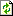Refresh page

Posted by Nick Gammon   Australia  (22,928 posts)bio   Forum Administrator
Date Sun 14 Jul 2013 01:50 AM (UTC)

Amended on Wed 07 Sep 2016 10:31 PM (UTC) by Nick Gammon

Message
 This post describes making a capacitor tester from an Arduino with minimal parts. Theory First, the theory is covered at some length by Martin Lorton here: How to measure a capacitor with an oscilloscope (YouTube) I decided to reproduce his test results myself. I used his test circuit: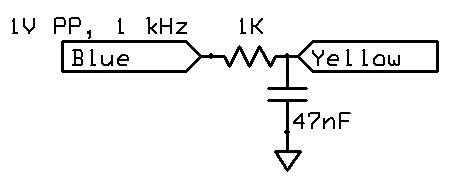Feeding in a 1 kHz signal (square wave) at 1V peak to peak, we see this: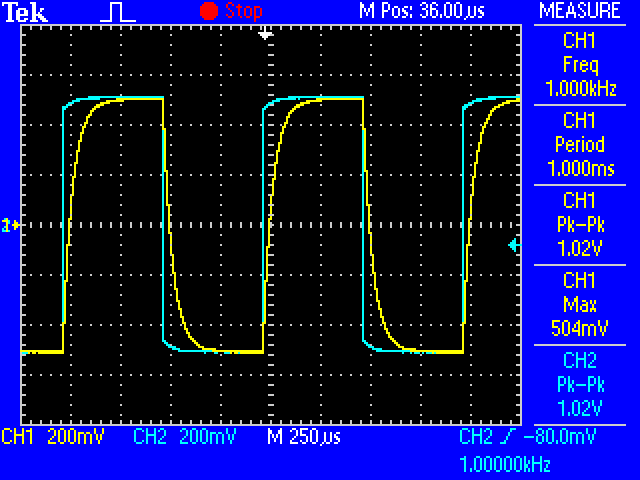Zooming in on one cycle, we find the point where the voltage reaches 63.2% (that is, 632 mV). We are choosing 63.2% because this is: `````` 1 - e^(-1) = 0.63212 `````` http://en.wikipedia.org/wiki/RC_time_constant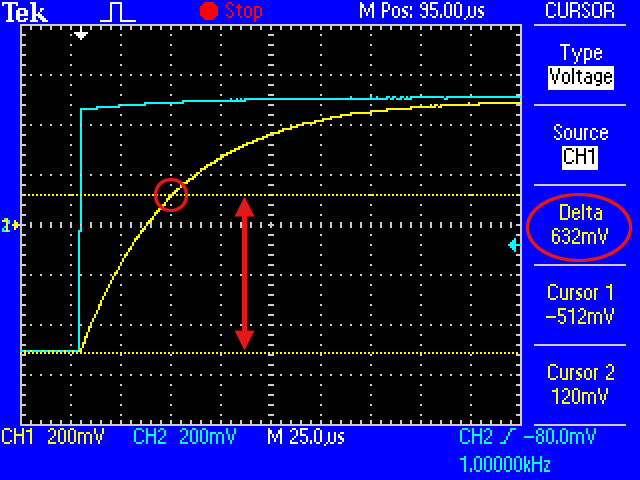Switching to the "time" cursor we measure how long it takes to charge to this point:From the Wikipedia article, we read: Quote:The RC time constant, the time constant (in seconds) of an RC circuit, is equal to the product of the circuit resistance(in ohms) and the circuit capacitance (in farads), i.e. τ = R * C. Thus C is equal to τ / R I measured 47 uS as the time, so thus the capacitance was: ``C = 0.000047 / 1000 `` In other words, 47 nF, which is in fact the capacitor I used. So far so good, the theory agrees with practice. Simple tester Circuit: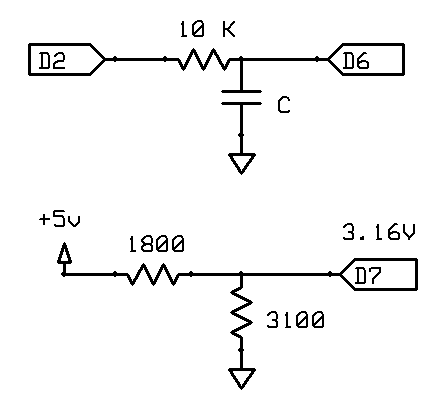The objective here is to find the time constant τ (tau) in the equation: `````` τ = R * C `````` Solved for C: `````` C = τ / R `````` So we want to charge the capacitor under test with a suitable voltage (eg. 5V from an Arduino output pin) and measure how long it takes to reach 63.2% of that voltage. So we need a reference voltage of 5 * 0.632, namely 3.16V. The voltage divider illustrated above should provide approximately that: Quote: Vout = Vin * (R2 / (R1 + R2)) `````` 5 * (3100 / (1800 + 3100)) = 3.163 `````` You may need to use a few resistors (eg. 3 x 1K plus 100 ohms) to make up the 3100 ohm resistor. We now need to time the interval indicated by the arrow and stop when the rising edge reaches the reference voltage:The internal analogue comparator is just the thing for the job. We connect the reference voltage to the AIN1 pin (negative reference) and connect our capacitor to the AIN0 pin (positive reference) and then configure an interrupt on the rising edge. I chose a value of 10K for the resistor to give a reasonably slow charge time. A simple sketch follows: `````` /* Capacitance meter Author: Nick Gammon Date: 27 June 2013 Pulse pin (D2): Connect to capacitor via 10K resistor. Reference voltage of 0.632 of output pin (pulsePin) connected to D7. In my case I used 3.06V because I measured 4.84 on the 5V pin. Measure pin (D6) connected to first leg of capacitor, other leg connected to Gnd. Like this: Capacitor to test: D2 ----> 10K ----> D6 ----> capacitor_under_test ----> Gnd Reference voltage: +5V ----> 1.8K ---> D7 ---> 3.1K ----> Gnd */ const byte pulsePin = 2; const unsigned long resistance = 10000; volatile boolean triggered; volatile boolean active; volatile unsigned long startTime; volatile unsigned long duration; ISR (ANALOG_COMP_vect) { // grab time quickly unsigned long now = micros (); if (active) { duration = now - startTime; triggered = true; digitalWrite (pulsePin, LOW); // start discharging capacitor } } void setup () { pinMode (pulsePin, OUTPUT); digitalWrite (pulsePin, LOW); Serial.begin (115200); Serial.println ("Started."); ADCSRB = 0; // (Disable) ACME: Analog Comparator Multiplexer Enable ACSR = bit (ACI) // (Clear) Analog Comparator Interrupt Flag | bit (ACIE) // Analog Comparator Interrupt Enable | bit (ACIS0) | bit (ACIS1); // ACIS1, ACIS0: Analog Comparator Interrupt Mode Select (trigger on rising edge) } // end of setup void loop () { // start another test? if (!active) { active = true; triggered = false; digitalWrite (pulsePin, HIGH); // start charging capacitor startTime = micros (); // remember when } // if the ISR noted the time interval is up, display results if (active && triggered) { active = false; Serial.print ("Capacitance = "); Serial.print (duration * 1000 / resistance); Serial.println (" nF"); triggered = false; delay (3000); } } // end of loop `````` With a 47 nF capacitor connected, I get this result: `````` Started. Capacitance = 47 nF Capacitance = 46 nF Capacitance = 47 nF Capacitance = 46 nF `````` Not too bad. But with a 6.8 nF capacitor we only get one decimal place: `````` Capacitance = 6 nF Capacitance = 7 nF Capacitance = 7 nF Capacitance = 7 nF Capacitance = 6 nF Capacitance = 6 nF Capacitance = 7 nF Capacitance = 6 nF Capacitance = 7 nF `````` Plus, the micros() function only returns time to the nearest 4 microseconds. Improved sketch using Timer 1 A slightly more elaborate sketch uses Timer 1 with a prescaler of 1 to get a higher resolution timer. `````` /* Capacitance meter Author: Nick Gammon Date: 27 June 2013 Pulse pin (D2): Connect to capacitor via 10K resistor. Reference voltage of 0.632 of output pin (pulsePin) connected to D7. In my case I used 3.06V because I measured 4.84 on the 5V pin. Measure pin (D6) connected to first leg of capacitor, other leg connected to Gnd. Like this: Capacitor to test: D2 ----> 10K ----> D6 ----> capacitor_under_test ----> Gnd Reference voltage: +5V ----> 1.8K ---> D7 ---> 3.1K ----> Gnd */ const byte pulsePin = 2; const float resistance = 10000.0; volatile boolean triggered; volatile boolean active; volatile unsigned long timerCounts; volatile unsigned long overflowCount; ISR (TIMER1_OVF_vect) { ++overflowCount; // count number of Counter1 overflows } // end of TIMER1_OVF_vect ISR (ANALOG_COMP_vect) { // grab counter value before it changes any more unsigned int timer1CounterValue; timer1CounterValue = TCNT1; // see datasheet, page 117 (accessing 16-bit registers) unsigned long overflowCopy = overflowCount; if (active) { // if just missed an overflow if ((TIFR1 & bit (TOV1)) && timer1CounterValue < 256) overflowCopy++; // calculate total count timerCounts = (overflowCopy << 16) + timer1CounterValue; // each overflow is 65536 more triggered = true; digitalWrite (pulsePin, LOW); // start discharging capacitor } } // end of ANALOG_COMP_vect void setup () { pinMode (pulsePin, OUTPUT); digitalWrite (pulsePin, LOW); Serial.begin (115200); Serial.println ("Started."); ADCSRB = 0; // (Disable) ACME: Analog Comparator Multiplexer Enable ACSR = bit (ACI) // (Clear) Analog Comparator Interrupt Flag | bit (ACIE) // Analog Comparator Interrupt Enable | bit (ACIS0) | bit (ACIS1); // ACIS1, ACIS0: Analog Comparator Interrupt Mode Select (trigger on rising edge) } // end of setup void startTiming () { active = true; triggered = false; // prepare timer overflowCount = 0; // no overflows yet // reset Timer 1 TCCR1A = 0; TCCR1B = 0; TCNT1 = 0; // Counter to zero TIFR1 = bit (TOV1); // clear overflow bit // Timer 1 - counts clock pulses TIMSK1 = bit (TOIE1); // interrupt on Timer 1 overflow // get on with it digitalWrite (pulsePin, HIGH); // start charging capacitor // start Timer 1, no prescaler TCCR1B = bit (CS10); } // end of startTiming void finishTiming () { active = false; Serial.print ("Capacitance = "); float capacitance = (float) timerCounts * 1000.0 / 16.0 / resistance; Serial.print (capacitance); Serial.println (" nF"); triggered = false; delay (3000); } // end of finishTiming void loop () { // start another test? if (!active) startTiming (); // if the ISR noted the time interval is up, display results if (active && triggered) finishTiming (); } // end of loop `````` Output for 6.8 nF capacitor: `````` Capacitance = 6.96 nF Capacitance = 6.96 nF Capacitance = 6.96 nF Capacitance = 6.95 nF Capacitance = 6.96 nF Capacitance = 6.97 nF `````` And the 47 nF capacitor looks good: `````` Capacitance = 46.94 nF Capacitance = 46.91 nF Capacitance = 46.94 nF Capacitance = 46.85 nF Capacitance = 46.91 nF Capacitance = 46.91 nF Capacitance = 46.91 nF `````` Improved sketch using the Input Capture Following on from a suggestion by "tmd3" on the Arduino forum, this next sketch uses the Input Capture feature of the timer to make a note of the timer value the moment the Analog Comparator makes a match. This preserves the exact count, so that it isn't out by the number of microseconds needed for the interrupt routine to get underway. At his suggestion we can do away with an exactly calculated voltage divider, and simply use two reasonable value resistors. We then plug those resistor values into the sketch and it works out "k" which is the multiplier to give us the capacitance based on the time taken to reach that point. The relevant lines are in bold below.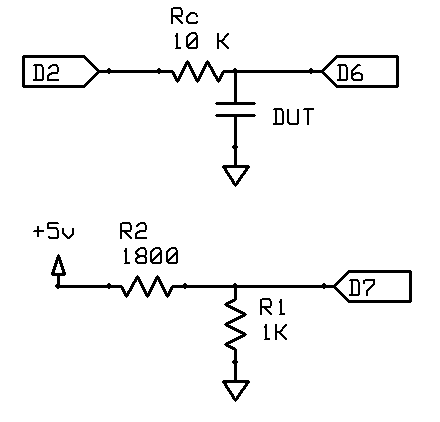(DUT = Device Under Test, namely the capacitor) `````` /* Capacitance meter Author: Nick Gammon Date: 2 July 2013 Pulse pin (D2): Connect to capacitor via 10K resistor (Rc) Reference voltage connected to D7 (AIN1) as per below. Measure pin (D6 - AIN0) connected to first leg of capacitor, other leg connected to Gnd. Like this: Capacitor to test: D2 ----> Rc ----> D6 ----> capacitor_under_test ----> Gnd Reference voltage: +5V ----> R2 ---> D7 ---> R1 ----> Gnd */ const byte pulsePin = 2; // the pin used to pulse the capacitor const float Rc = 10000; // charging resistor const float R1 = 1000; // between ground and D7 const float R2 = 1800; // between +5V and D7 const float clockRate_us = 16; // 16 MHz const float k = 1000 / (clockRate_us * Rc * log ((R1 + R2) / R2)); volatile boolean triggered; volatile boolean active; volatile unsigned long timerCounts; volatile unsigned long overflowCount; ISR (TIMER1_OVF_vect) { ++overflowCount; // count number of Counter 1 overflows } // end of TIMER1_OVF_vect ISR (TIMER1_CAPT_vect) { // grab counter value before it changes any more unsigned int timer1CounterValue; timer1CounterValue = ICR1; // see datasheet, page 117 (accessing 16-bit registers) unsigned long overflowCopy = overflowCount; if (active) { // if just missed an overflow if ((TIFR1 & bit (TOV1)) && timer1CounterValue < 0x7FFF) overflowCopy++; // calculate total count timerCounts = (overflowCopy << 16) + timer1CounterValue; // each overflow is 65536 more triggered = true; digitalWrite (pulsePin, LOW); // start discharging capacitor TCCR1B = 0; // stop the timer } // end if active } // end of TIMER1_CAPT_vect void setup () { pinMode (pulsePin, OUTPUT); digitalWrite (pulsePin, LOW); Serial.begin (115200); Serial.println ("Started."); ADCSRB = 0; // (Disable) ACME: Analog Comparator Multiplexer Enable ACSR = bit (ACIC); // Analog Comparator Input Capture Enable DIDR1 |= bit (AIN1D) | bit (AIN0D); // Disable digital buffer on comparator inputs PRR = 0; } // end of setup void startTiming () { active = true; triggered = false; noInterrupts (); // prepare timer overflowCount = 0; // no overflows yet // reset Timer 1 TCCR1A = 0; TCCR1B = 0; TCNT1 = 0; // Counter to zero // Timer 1 - counts clock pulses TIMSK1 = bit (TOIE1) | bit (ICIE1); // interrupt on Timer 1 overflow and input capture // get on with it digitalWrite (pulsePin, HIGH); // start charging capacitor // start Timer 1, no prescaler TCCR1B = bit (CS10) | bit (ICES1); // plus Input Capture Edge Select interrupts (); } // end of startTiming void finishTiming () { active = false; Serial.print ("Capacitance = "); float capacitance = (float) timerCounts * k; Serial.print (capacitance); Serial.println (" nF"); triggered = false; delay (3000); } // end of finishTiming void loop () { // start another test? if (!active) startTiming (); // if the ISR noted the time interval is up, display results if (active && triggered) finishTiming (); } // end of loop `````` Discussion Discussion about this project on these Arduino forum threads: Measuring capacitance with an oscilloscope Turn your Arduino into a capacitor tester ... - Nick Gammon www.gammon.com.au, www.mushclient.com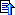top

The dates and times for posts above are shown in Universal Co-ordinated Time (UTC).

To show them in your local time you can join the forum, and then set the 'time correction' field in your profile to the number of hours difference between your location and UTC time.

25,304 views.Refresh page

Go to topic:           Search the forumtop

Quick links: MUSHclient. MUSHclient help. Forum shortcuts. Posting templates. Lua modules. Lua documentation.

Information and images on this site are licensed under the Creative Commons Attribution 3.0 Australia License unless stated otherwise.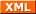Forum RSS feed ( https://gammon.com.au/rss/forum.xml )